• Python实现信号滤波（基于scipy)
千次阅读
2021-09-11 14:26:56

### 作用：去除干扰信号

1. 低通滤波：去除高于某一阈值频率的信号；'lowpass'

2. 高通滤波：去除低于某一频率的信号；'highpass'

3. 带通滤波：类似低通高通的结合保留中间频率信号；'bandpass'

4. 带阻滤波器：低通高通的结合只是过滤掉的是中间部分；'bandstop'

一、滤波器构造函数:scipy.signal.butter(N, Wn, btype='low', analog=False, output='ba')

N:滤波器的阶数

Wn：归一化截止频率(计算公式Wn=2*截止频率/采样频率)

btype : 滤波器类型{‘lowpass’, ‘highpass’, ‘bandpass’, ‘bandstop’}

output : 输出类型{‘ba’, ‘zpk’, ‘sos’}

二、滤波函数：scipy.signal.lfilter(b, a, x, axis=-1, zi=None)

使用IIR或FIR过滤器沿one-dimension过滤数据

举例代码：

from scipy import signal

b, a = signal.butter(8, 0.2, 'highpass')   #配置滤波器 8 表示滤波器的阶数

filtedData = signal.filtfilt(b, a, data)   #data为要过滤的信号python
更多相关内容
• 本文将以实战的形式基于scipy模块使用Python实现简单滤波处理。这篇文章主要介绍了Python基于scipy实现信号滤波功能,需要的朋友可以参考下
• 信号滤波处理。。。。。。。。。。。。。。。。。。。。。。。。。。。。。。。。。。。。
• 简单的理解就是低通滤波指的是去除高于某一阈值频率的信号；高通滤波去除低于某一频率的信号；带通滤波指的是类似低通高通的结合保留中间频率信号；带阻滤波也是低通高通的结合只是过滤掉的是中间部分。上面所说的...
• 主要编写了移动平均滤波器、低通滤波器、巴特沃斯低通滤波对经过信号之后的滤波效果
• labview小程序，用于处理采集到的数据对其滤波并求信号频率
• 本文介绍一套用于轧线 PLC 控制的模拟量信号采样、滤波的方法。实现了自由灵活的信号处理。信号稳定性、可靠性均较滤波前有较大的改善。在轧线张力控制环节中滤波效果显着，满足了生产的要求。此功能经模块化编程后...
• 关于数字信号处理时的算法参考资料-中值与小波消噪集成的转子振动信号滤波方法研究.pdf 关于数字信号处理时的算法参考资料，希望可以给大家一点帮助！！！matlab
• 心电信号的处理，包括低通滤波器滤除肌电信号，带陷滤波器抑制工频干扰，以及IIR零相移数字滤波器纠正基线漂移。
• 锋电位信号是利用微电极阵列采集的大鼠海马区神经元信号．结果表明，下限截止频率小于100 Hz且上限截止频率大于5 000 Hz时，滤波造成的锋电位波形失真较小．对于锋电位的检出和分类这2种数据处理，它们的上限截止...
• 音频信号滤除噪声，恢复原信号。。。。。。。。。。。。。。。。。。。
• ## Matlab 实现信号滤波

万次阅读 多人点赞 2020-11-30 10:07:26
文章目录项目介绍代码实现1、导入信号2、加入噪声3、绘制原始信号的时域、频域4、滤波4.1 移动平均滤波4.2 中值滤波4.3 维纳滤波4.4 自适应滤波4.5 巴特沃斯滤波4.5.1 低通滤波4.5.2 高通滤波4.5.3 带通滤波 ...

# 项目介绍

在此文章中，信号的导入、滤波以及时频转换将被介绍。

# 代码实现

## 1、导入信号

这里我们使用三种不同频率的信号的叠加信号。

clc
clear

fs = 1000;  % 重采样频率
T = 1/fs;  % 周期
n = 5;  % 1Hz频率被分成n段
N = fs*n;  % 因为1Hz频率被分成了n段，所以频谱的x轴数组有fs*n个数
f = (0: N-1)*fs/N;  % 将fs个频率细分成fs*n个（即原来是[0, 1, 2, …, fs]，现在是[0, 1/N, 2/N, …, (N-1)*fs/N]）
t = (0: N-1)*T;  % 信号所持续的时长（N个周期）
nHz = 10;  % 画的频谱的横坐标到nHz
Hz = nHz*n;  % 画的频谱的横坐标的数组个数

x_ori = sin(2*pi*5*t)+0.7*sin(2*pi*2*t)+0.4*sin(2*pi*7*t-1);


## 2、加入噪声

Noise_White = [0.3*randn(1,length(t)/2), rand(1,length(t)/2)];  % 加入白噪声
x = x_ori + Noise_White;


## 3、绘制原始信号的时域、频域

figure
subplot(211),plot(x,'k'),title('原始信号时域'),xlabel('time [s]');  % 绘制原始信号时域
fx = abs(fft(x-mean(x)))/(N/2);  % 傅里叶变换
subplot(212),plot(f(1:Hz), fx(1:Hz),'k'),title('原始信号频域'),xlabel('frequency [Hz]');  % 绘制原始信号频域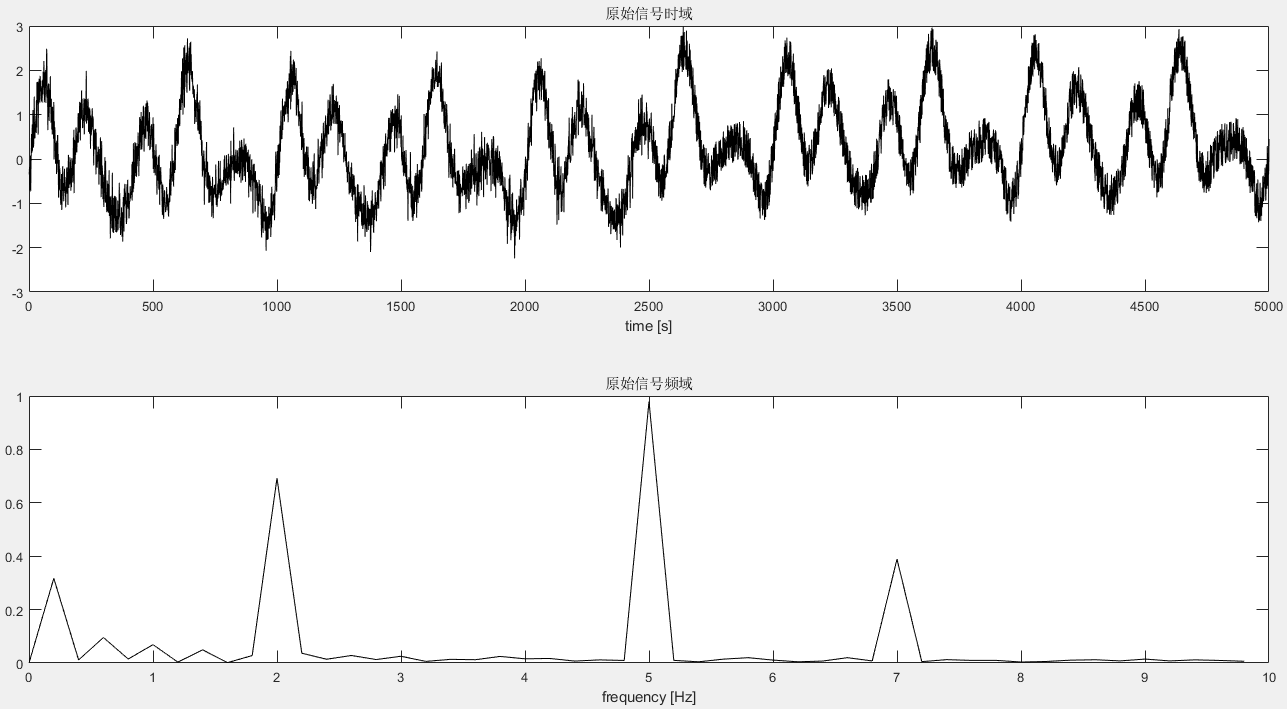由频域图可以看出该信号有三个主频率成分，分别为2Hz、5Hz以及7Hz。

## 4、滤波

### 4.1 移动平均滤波

b  =  [1 1 1 1 1 1]/6;
x1 = filter(b,1,x);

figure
subplot(211),plot(x1,'b'),title('滤波后的信号'),xlabel('time [s]');
fx1 = abs(fft(x1-mean(x1)))/(N/2);  % 傅里叶变换
subplot(212),plot(f(1:Hz), fx1(1:Hz),'k'),title('滤波信号频域'),xlabel('frequency [Hz]');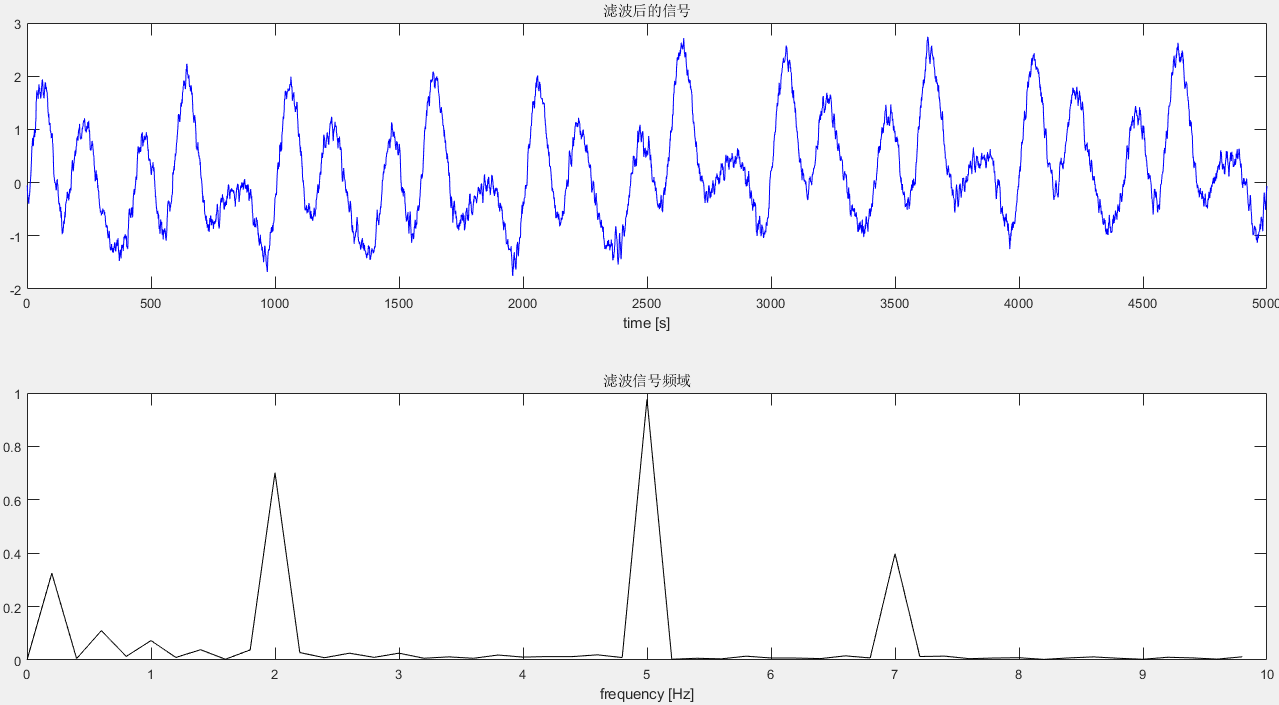### 4.2 中值滤波

x1=medfilt1(x,10);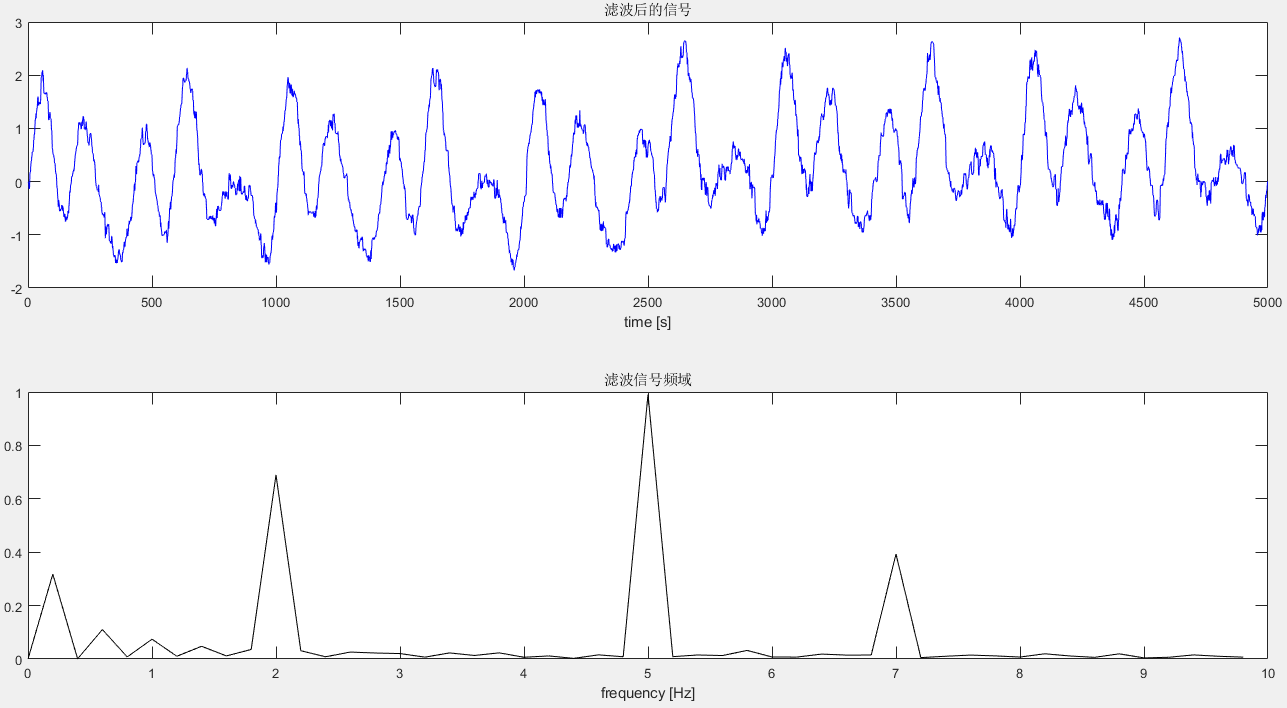### 4.3 维纳滤波

Rxx=xcorr(x, x);              %得到混合信号的自相关函数
M=100;                                                             %维纳滤波器阶数
for i=1:M
for j=1:M
rxx(i,j)=Rxx(abs(j-i)+N);   %得到混合信号的自相关矩阵
end
end
Rxy=xcorr(x,x_ori);       %得到混合信号和原信号的互相关函数
for i=1:M
rxy(i)=Rxy(i+N-1);   %得到混合信号和原信号的互相关向量
end
h = inv(rxx)*rxy';                                               %得到所要涉及的wiener滤波器系数
x1=filter(h,1, x);               %将输入信号通过维纳滤波器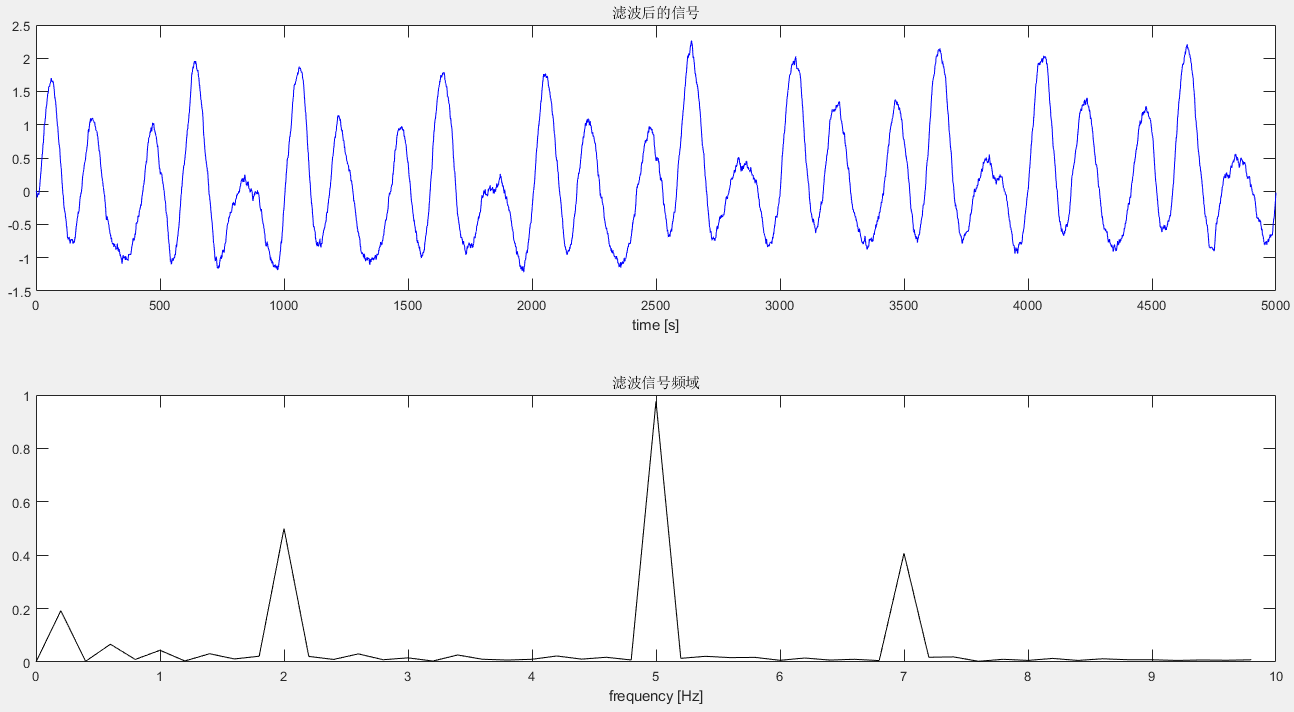### 4.4 自适应滤波

k=100;                                                  %时域抽头LMS算法滤波器阶数
u=0.001;                                             %步长因子

%设置初值
x1=zeros(1,N);                                  %output signal
w=zeros(1,k);                                        %设置抽头加权初值
e=zeros(1,N);                                        %误差信号

%用LMS算法迭代滤波
for i=(k+1):N
XN=x((i-k+1):(i));
x1(i)=w*XN';
e(i)=x_ori(i)-x1(i);
w=w+2*u*e(i)*XN;
end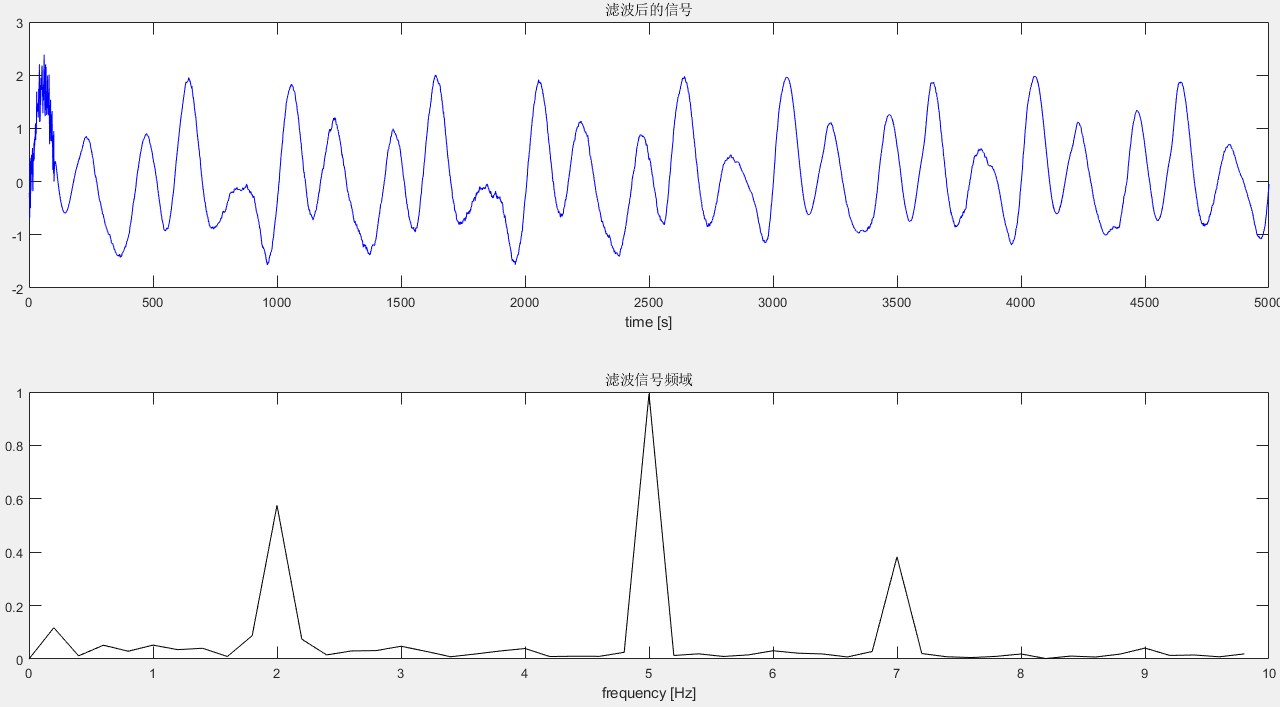### 4.5 巴特沃斯滤波

#### 4.5.1 低通滤波

Wc=2*3/fs;                                          %截止频率 3Hz
[b,a]=butter(4,Wc,'low');  % 四阶的巴特沃斯低通滤波
x1=filter(b,a,x);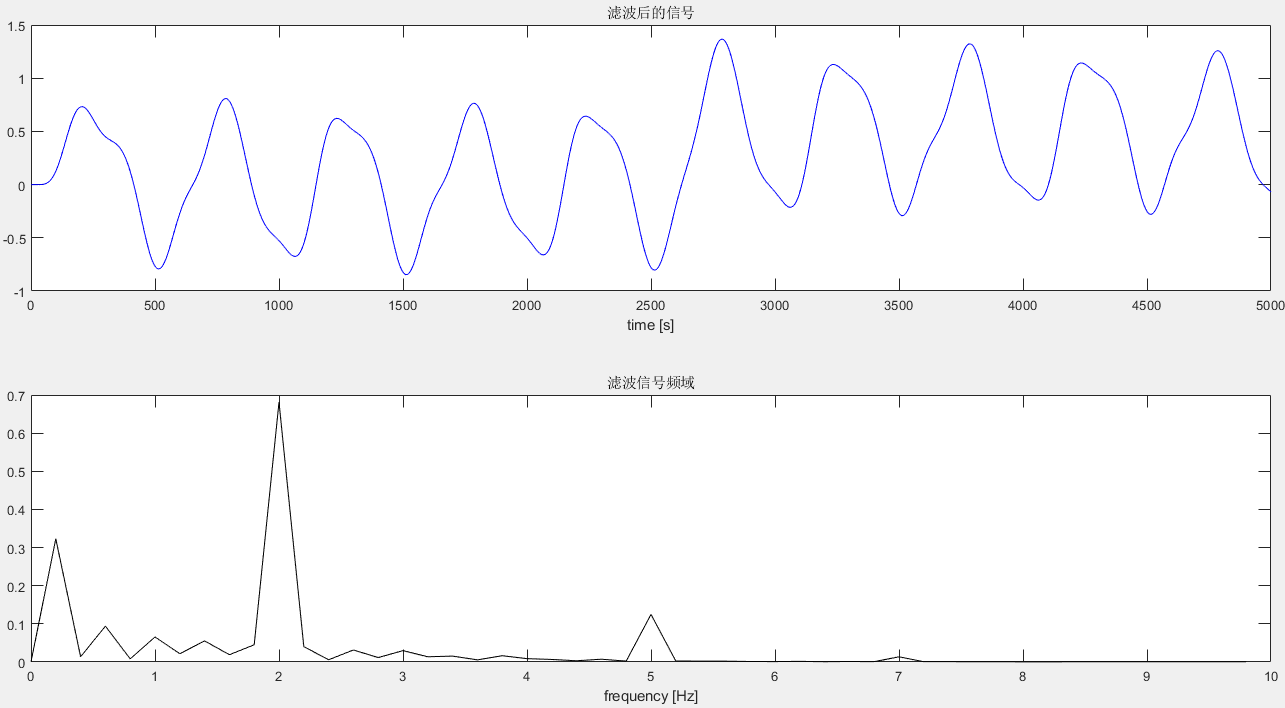由图可见，3Hz以上的信号幅值明显降低。

#### 4.5.2 高通滤波

Wc=2*3/fs;                                          %截止频率 3Hz
[b,a]=butter(4,Wc,'high');  % 四阶的巴特沃斯高通滤波
x1=filter(b,a,x);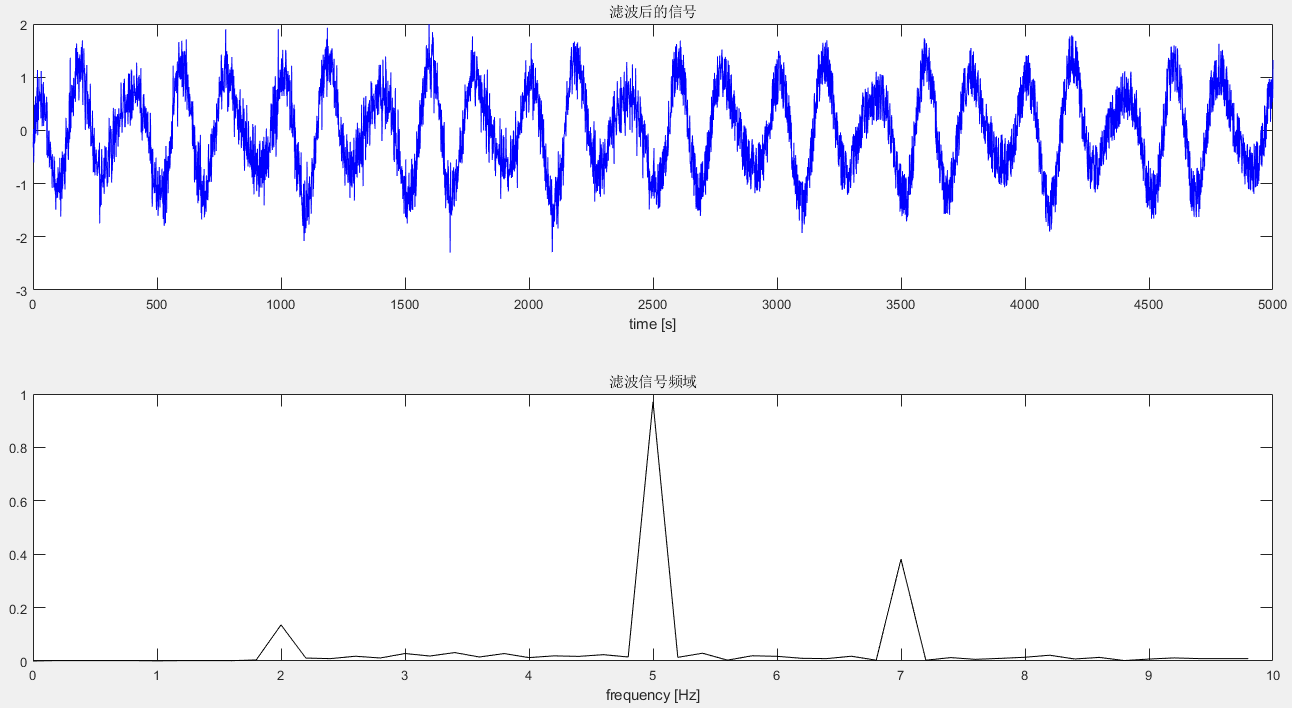由图可见，3Hz以下的信号幅值明显降低。

#### 4.5.3 带通滤波

Wc1=2*1/fs;                                          %下截止频率 1Hz
Wc2=2*6/fs;                                          %上截止频率 6Hz
[b,a]=butter(2,[Wc1, Wc2],'bandpass');  % 二阶的巴特沃斯带通滤波
x1=filter(b,a,x);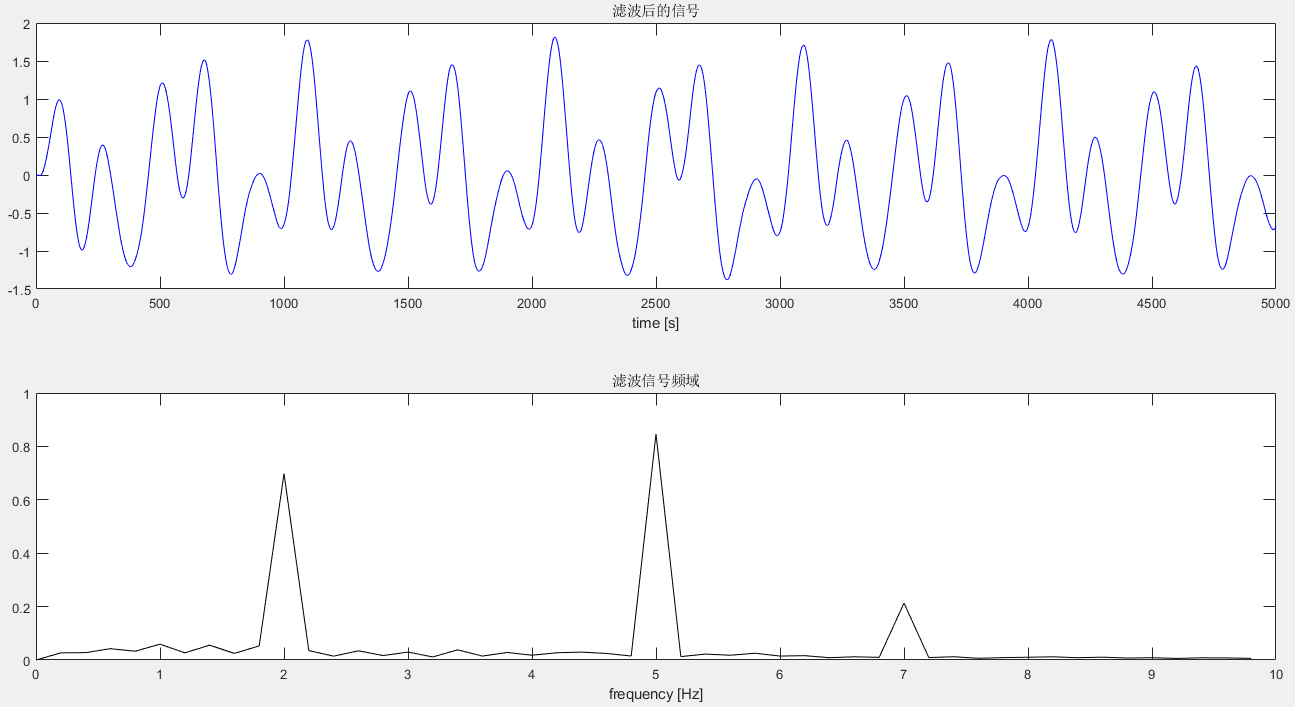由图可见，1Hz到6Hz以外的信号幅值明显降低。

展开全文matlab filter
• 基于matlab的语音信号滤波处理摘要：本课程设计的主要目的是在MATLAB环境下，使用窗口设计法设计一个滤波器，并对语音信号进行滤波去噪。开发平台为MATLAB，设计方法为窗口设计法。用麦克风采集一段语音信号，绘制...

基于matlab的语音信号滤波处理

摘要：本课程设计的主要目的是在MATLAB环境下，使用窗口设计法设计一个滤波器，并对语音信号进行滤波去噪。开发平台为MATLAB，设计方法为窗口设计法。用麦克风采集一段语音信号，绘制波形并观察其频谱，给定相应技术指标，用凯塞窗设计一个满足指标的FIR滤波器，对该语音信号进行滤波去噪处理，比较滤波前后的波形和频谱并进行分析。在滤波前后语音信号波形的变化中，由于我们录制的语音信号噪声不大，所以观察并不明显，但在频域波形中，我们可以明显的看到设计的滤波器对语音信号进行了滤波处理，将噪声进行了滤除。此次滤波基本达到了要求，完成了设计指标。

关键词：滤波去噪；FIR滤波器；凯塞窗；MATLAB

目录

1 课程设计研究内容 1

1.1课程设计研究内容 1

1.2课程设计步骤及流程图 1

1.3课程设计要求 3

2 设计原理 3

2.1 MATLAB简介 3

2.2 滤波器 3

3 设计与实现过程 3

3.1实现方法 3

3.1.1 FIR滤波器 4

3.1.2窗口设计法 4

3.1.3凯塞窗 4

3.2 设计过程 4

3.2.1录制语音信号 4

3.2.2 对原信号加入噪声 5

3.2.2 滤波器的设计 7

3.2.2信号的滤波处理 9

3.3 仿真结果与分析 10

4 调试问题与解决方案 11

5总结与展望 11

致谢 12

参考文献 12

附录 12

附录1. 滤波器脉冲响应源程序 12

附录2. 理想低通滤波器计算源程序 13

## 1.1设计研究内容

1.语音信号的采集

2.语音信号的频谱分析

用MATLAB程序对原始语音信号进行采样、频谱分析，并绘制出采样后语言信号时域波形图和频谱图，并针对此图分析语音信号特点。

3.语音信号加噪与频谱分析

利用MATLAB程序产生信号噪声，并加入到语音信号中，模仿语音信号被污染，并对其频谱分析，与原始语音信号进行对比，分析差异。

4.设计数字滤波器

根据语音信号的特点，设计数字滤波器，对加噪后的语音信号进行滤波处理。

5.验证滤波器的滤波效果

对滤波后的语音信号进行时域、频域分析，并将滤波前后的时域波形、频谱波形进行相比较，分析信号的变化，从而验证所设计滤波器的滤波效果是否达到了滤除高频噪音、保留低频原始语音信号的目的。

6.回放语音信号

利用函数sound对滤波后语音信号进行回放。

## 1.2设计步骤及流程图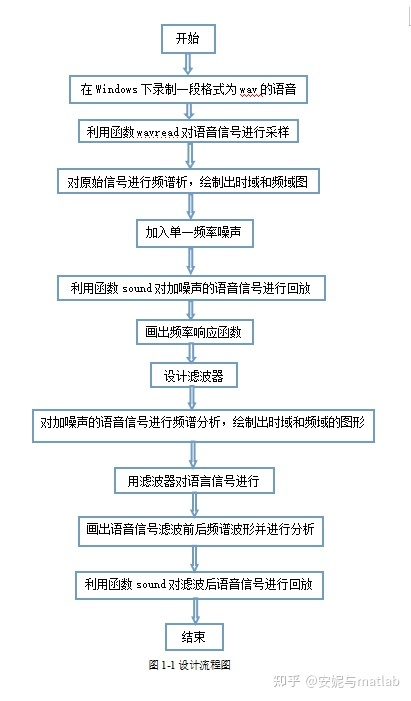## 2.1 MATLAB简介

MATLAB是一个为科学和工程计算专门设计的交互式大型软件，是一个可以完成各种精确计算和数据处理的，可视化的，强大的计算工具。它具有丰富的函数资源和工具箱资源，语言精练，代码灵活，面向对象，控制功能优良，图形工能也强大。并且它的兼容性很好，几乎能在所有的PC机和大型计算机上运行，适用于Windows，UNIX和多种系统平台。MATLAB形形色色的工具箱中包括控制系统，信号处理，小波分析，统计，优化等，能够很好的运用于语音信号的滤波去噪。

## 2.2 滤波器

数字滤波器在数字信号处理的各种应用中发挥着十分重要的作用。它是通过对采样数据信号进行数学运算处理来达到滤波的目的。FIR数字滤波器设计的方法有三类，一类是窗口设计法（时间窗口法），第二类是频率采样法，第三类是等波纹优化设计。时间窗口设计法是从单位脉冲响应序列着手，使h(n)逼近理想的单位脉冲响应序列hd(n)；频率采样法是使所设计的FIR数字滤波器的频率特性某些离散频率点上的值准确地等于所需滤波器在这些频率点处的值，在其它频率处的特性则有较好的逼近。等波纹优化设计也叫最佳一致逼近准则，最佳一致逼近即选择N个频率采样值（或时域h(n) 值），在给定频带范围内使频响的最大逼近误差达到最小。可保证局部频率点的性能也是最优的，误差分布均匀，相同指标下，可用最少的阶数达到最佳化。本次课程设计采用的就是窗口设计法。

## 3.1实现方法

3.1.1 FIR滤波器

数字滤波器(Digital Filter，简称为DF)是指用来对输入信号进行滤波的硬件和软件。 所谓数字滤波器，是指输入、输出均为数字信号，通过一定运算关系改变输入信号所含频率成分的器件。数字滤波器和模拟滤波器相比，因为信号的形式和实现滤波的方法不同，数字滤波器具有比模拟滤波器精度高、稳定、体积小、重量轻、灵活、不要求阻抗匹配等优点。一般用两种方法来实现数字滤波器:一是采用通用计算机，把滤波器所要完成的运算编成程序通过计算机来执行，也就是采用计算机软件来实现;二是采用实际专用的数字处理硬件。

数字滤波器按照冲激响应的时域特性可分为:无限长单位冲响应滤波器（IIR）和有限长单位冲击响应滤波器（FIR），但与IIR相比，在满足同样阻带衰减的情况下需要的阶数较高，滤波器的阶数越高，占用的运算时间就越多，因此在满足指标要求的情况下应尽量减少滤波器的阶数。

FIR滤波器的基本结构可以理解为一个分节的延时线，把每一节的输出加权累加，可得到滤波器的输出，FIR滤波器的冲激响应h(n)是有限长的，数学M阶FIR滤波器可以表示为：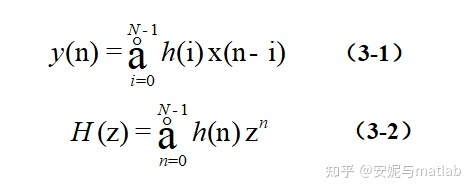3.1.2窗口设计法

窗口设计法的基本思想是要选取某一种合适的理想频率选择性滤波器（这种滤波器总是有一个非因果，无限长的脉冲响应），然后将它的脉冲响应截断（或加窗）以得到一个线性相位和因果的FIR滤波器。

3.1.3凯塞窗

窗函数的主瓣宽度和旁瓣峰值衰耗是矛盾的，一项指标的提高总是以另一项指标的下降为代价，窗口选择实际上是对两项指标作权衡。而两项指标是跳变的，于是有人提出可调整窗，适当修改参数，可在这两项指标间作连续的选择。常用的可调整窗是凯塞（Kaiser）窗。凯塞（Kaiser）窗全面地反映主瓣与旁瓣衰减之间的交换关系， 可以在它们两者之间自由地选择它们的比重。

凯塞窗的表达式是：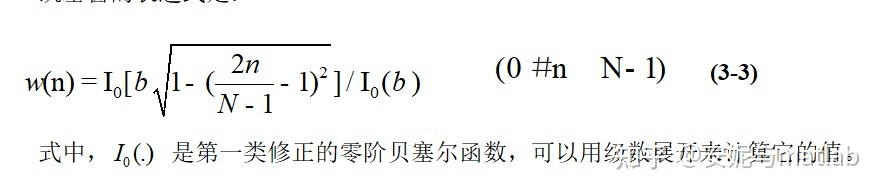## 3.2 设计过程

3.2.1录制语音信号

sound(x,fs,bits); % 按指定的采样率和每样本编码位数回放录音

x=x(:,2);

N=length(x); % 计算信号x的长度

fn=1000; % 单频噪声频率

t=0:1/fs:(N-1)/fs; % 计算时间范围，样本数除以采样频率

x=x';

y=x+0.1.*sin(fn*2*pi*t); %y为加入单频干扰信号后的语音

sound(y,fs,bits) ;

在MATLAB平台上，用plot函数画出原始语音信号，如图3-1所示：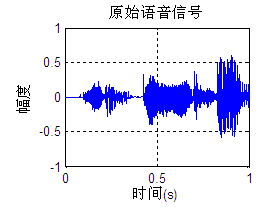3.2.2 对原信号加入噪声

在MATLAB平台上，对原始信号和加噪信号进行fft变换,取幅度谱,并对频谱进行分析。具体实现如下：

sound(x,fs,bits); % 按指定的采样率和每样本编码位数回放录音

x=x(:,2);

N=length(x); % 计算信号x的长度

fn=1000; % 单频噪声频率

t=0:1/fs:(N-1)/fs; % 计算时间范围，样本数除以采样频率

x=x';

y=x+0.1.*sin(fn*2*pi*t); %y为加入单频干扰信号后的语音

sound(y,fs,bits) ; % 对加噪信号进行回放

X=abs(fft(x));% 对原始信号进行fft变换,取幅度谱

Y=abs(fft(y)); % 对加噪后信号进行fft变换,取幅度谱

X=X(1:N/2); Y=Y(1:N/2); % 截取前半部分

f=0:deltaf:fs/2-deltaf; % 计算频谱频率范围

subplot(2,2,1);plot(t,x); axis([0 1 -1 1]); grid on; %画原始语音信号的时域图

xlabel('时间(s)');ylabel('幅度');title('原始语音信号');

subplot(2,2,2);plot(f,X);axis([0 4000 0 1500]); grid on; %画原始语音信号幅度谱图

xlabel('频率(Hz)');ylabel('幅度谱');title('语音信号幅度谱图');

subplot(2,2,3);plot(t,y); axis([0 1 -1 1]); grid on; %画加噪语音信号的时域图

xlabel('时间(s)');ylabel('幅度');title('加入单频干扰后的语音信号');

subplot(2,2,4);plot(f,Y);axis([0 4000 0 1500]); grid on; %画加噪语音信号幅度谱图

xlabel('频率(Hz)');ylabel('幅度谱');title('加入干扰后的语音信号幅度谱图');

在MATLAB上运行结果如图3-2所示：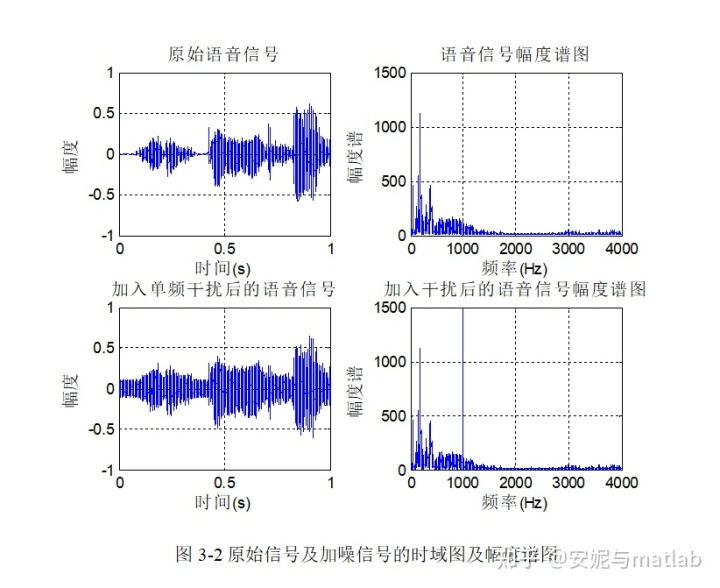由图3-2可以看出，对比原始信号与加噪信

号幅度谱图我们可以清晰的看到在1000Hz是出现了一个脉冲，从而使原有的音频听上去有了噪声，这样就到达了预期结果

3.2.2 滤波器的设计

滤波器设计就是要找到一组能满足特定滤波要求的系数向量a和b，而它主要是通过设计指标来实现的。滤波器设计的要求或指标一般是在频域上给出的，常用的滤波器频域指标有：fp1、fs1、fs2、fp2、Rp、As。要达到最佳的滤波效果，则需要对fp1、fs1、fs2、fp2和Rp、As进行适当的调整。由图3-3可以看出，语音信号可以选择fp1=900；fs1=950；fs2=1050；fp2=1100；Rp=1；As=60的滤波器。在MATLAB中，通常采用1/2采样频率进行归一化处理，如果将频率转化为角频率，则需将归一化频率乘以pi。设计程序如下：

fp1=900;fs1=950;fs2=1050;fp2=1100;Rp=1;As=60; % 带阻滤波器设计指标

fc1=(fp1+fs1)/2;fc2=(fp2+fs2)/2; df=min((fs1-fp1),(fp2-fs2)); % 计算上下边带中心频率，和频率间隔

ws1=fs1/fs*2*pi;ws2=fs2/fs*2*pi;

M=ceil((As-7.95)/(2.285* dw)+1)+1; % 计算汉宁窗设计该滤波器时需要的阶数

n=0:M-1; beta=0.1102*(As-8.7); % 定义时间范围

w_kaiser= kaiser(M,beta); % 产生M阶的凯塞窗

hd_bs=ideal_lp(wc1,M)+ideal_lp(pi,M)-ideal_lp(wc2,M); % 调用自编函数计算理想带阻滤波器的脉冲响应

h_bs=w_kaiser'.*hd_bs; % 用窗口法计算实际滤波器脉冲响应

[db,mag,pha,grd,w]=freqz_m(h_bs,1); % 调用自编函数计算滤波器的频率特性

Rp=-min(db(wc1/dw+1:1:wc2/dw)); As=max(-round(db(ws2/dw+1:1:501)));

subplot(2,2,1);plot(w/pi,db);axis([0 0.2 -70 10]);

xlabel('w/pi');ylabel('dB');title('滤波器幅度响应图');grid on;

subplot(2,2,2);plot(w/pi,mag);axis([0 0.2 0 1.2]);

xlabel('w/pi');ylabel('幅度mag');title('滤波器幅度响应图');grid on;

subplot(2,2,3);plot(w/pi,pha);axis([0 1 -3 3]);

xlabel('w/pi');ylabel('相位pha');title('滤波器相位响应图');grid on;

subplot(2,2,4);stem(n,h_bs);axis([0 1500 0 1]);

xlabel('n');ylabel('h(n)');title('滤波器脉冲响应图');grid on;

所设计的滤波器的幅度响应和脉冲响应如图3-3所示：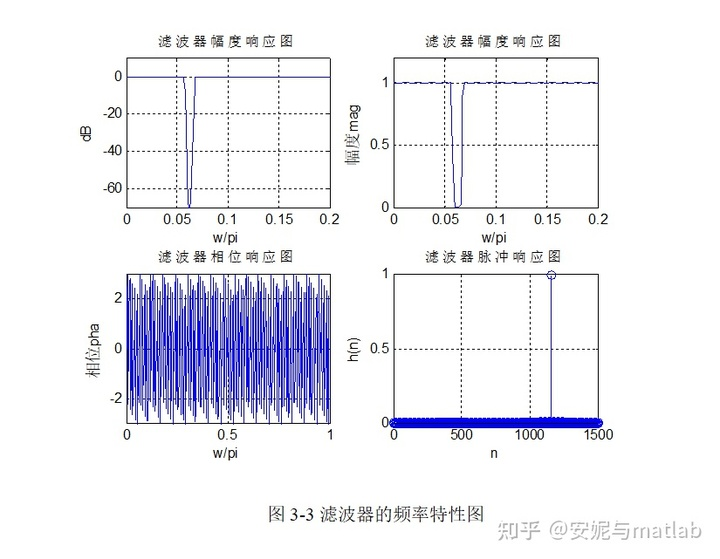由3-3图可以看出Rp接近于0，而As大于开始的设定值（As=60db)，由此可见满足了设计要求，达到了设计目的。

3.2.2信号的滤波处理

滤波器设计完成后，在MATLAB平台上调用函数fftfilt对信号实现滤波，绘制语音信号去噪前后时域图，频域幅度图形并进行比较。具体程序如下：

y_fil=fftfilt (h_bs, y); % 用设计好的滤波器对y进行滤波

Y_fil=fft(y_fil);Y_fil=Y_fil(1:N/2); % 计算频谱取前一半

subplot(3,2,1);plot(t,x); axis([0 3 -0.2 0.2]);

xlabel('时间(s)');ylabel('幅度');title('原始语音信号x');grid on;

subplot(3,2,2);plot(f,X);axis([0 4000 0 70]);

xlabel('频率(Hz)');ylabel('幅度谱');title('语音信号幅度谱X');grid on;

subplot(3,2,3);plot(t,y); axis([0 3 -0.2 0.2]);

xlabel('时间(s)');ylabel('幅度');title('加干扰后的语音信号x1');grid on;

subplot(3,2,4);plot(f,Y);axis([0 4000 0 70]);

xlabel('频率(Hz)');ylabel('幅度谱');title('加干扰语音信号幅度谱X1');grid on;

subplot(3,2,5);plot(t,y_fil);axis([0 3 -0.2 0.2])

xlabel('时间(s)');ylabel('幅度');title('滤波后语音信号y');grid on;

subplot(3,2,6);plot(f,Y_fil); axis([0 4000 0 70])

xlabel('频率(Hz)');ylabel('幅度谱');title('滤波后语音信号幅度谱Y');grid on;

sound (y_fil,fs,bits)

在MATLAB平台上的运行结果如图3-4所示：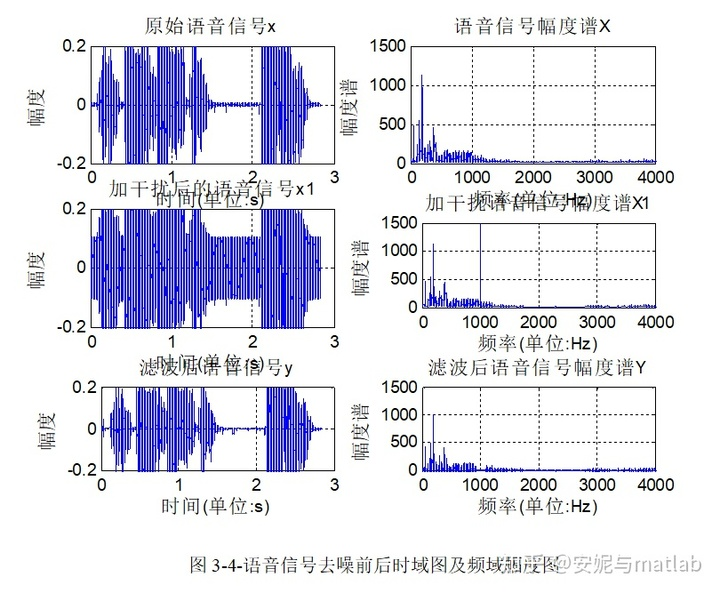由图3-4可以看出，在原始信号上加入的1000Hz 的脉冲信号已经被设计的滤波器滤去，符合实验所要到达的预期效果。

## 3.3 仿真结果与分析

通过观察滤波前后语音信号波形的变化，即观察图3-3，我们可以知道，原信号的时域图与滤波去噪信号的时域图基本相似；原信号与滤波去噪信号的频谱图波形也大致相似。通过观察可以看到，加噪信号的时域图中大部分都被加入的噪声给遮盖了，加噪信号的频谱图中，我们可以很明显地看到与原信号频谱图相比，它在频率1000Hz的地 方有一个尖脉冲，而滤波去噪信号的频谱图中该尖脉冲已经消失，幅度变为了零，波形大致与原图相似，可见滤波去噪效果基本不错。在将三个信号的时域波形和频谱图比较之后，我们还要通过回放去滤波去噪音乐信号，来跟原信号相比，以检验滤波器的效果。在MATLAB中，函数sound可以对声音进行回放。其调用格式为：sound (x，fs，bits)。用sound(y_fil,fs,bits)语句回放该滤波去噪信号，便可以感觉到滤波后的语音号与原信号差不多。

## 附录1. 滤波器脉冲响应源程序

function [db,mag,pha,grd,w] = freqz_m(b,a);

% freqz 子程序的改进版本

% ------------------------------------

% [db,mag,pha,grd,w] = freqz_m(b,a);

% db = [0 到pi弧度]区间内的相对振幅(db)

% mag = [0 到pi弧度]区间内的绝对振幅

% pha = [0 到pi弧度]区间内的相位响应

% grd = [0 到pi弧度]区间内的群迟延

% w = [0 到pi弧度]区间内501个频率样本向量

% b = Ha(z)的分子多项式系数(对FIR b=h)

% a = Ha(z)的分母多项式系数(对FIR: a=)

%

[H,w] = freqz(b,a,1000,'whole');

H = (H(1:1:501))'; w = (w(1:1:501))';

mag = abs(H);

db = 20*log10((mag+eps)/max(mag));

pha = angle(H);

% pha = unwrap(angle(H));

grd = grpdelay(b,a,w);

% grd = diff(pha);

% grd = [grd(1) grd];

% grd = [0 grd(1:1:500); grd; grd(2:1:501) 0];

% grd = median(grd)*500/pi;

## 附录2. 理想低通滤波器计算源程序

function hd = ideal_lp(wc,M);

% 理想低通滤波器计算

% --------------------------------

% [hd] = ideal_lp(wc,M)

% hd = 0 to M-1之间的理想脉冲响应

% wc = 截止频率(弧度)

% M = 理想滤波器的长度

%

alpha = (M-1)/2;

n = [0:1:(M-1)];

m = n - alpha + eps;

hd = sin(wc*m) ./ (pi*m);

展开全文python 算法 matlab 机器学习
• 对于噪声中的信号检测，通常会涉及三个方面：信号滤波、信号判决、信号参量估计。信号滤波是信号处理中经常采用的方法，主要是从被噪声污染的信号中恢复信号波形；信号判决主要是解决从强干扰噪声中发现和分辨微弱...

对于噪声中的信号检测，通常会涉及三个方面：信号滤波、信号判决、信号参量估计。信号滤波是信号处理中经常采用的方法，主要是从被噪声污染的信号中恢复信号波形；信号判决主要是解决从强干扰噪声中发现和分辨微弱信号的问题，即判断噪声中有无信号的问题；信号参量估计是关于噪声中信号未知参量的测量问题，是在肯定信号存在的情况下计算信号的参数。

微弱信号滤波问题：
1.匹配滤波器
2.维纳滤波器
3.卡尔曼滤波器
4.自适应噪声抵消滤波器（希望滤波器能够自动适应系统或周围环境的变化，则需要滤波器的参数可以随时间做些简单的变化。）

在设计滤波器时，应采用某些准则，使最终的采集到的信号满足人们的需求；通常采用两种准则：一种是使滤波器输出的信号的信噪比(SNR)达到最大；另一种是使用滤波器输出信号的均方误差最小。采用第一种准则的称为匹配滤波器；采用第二种准则的称为维纳(Wiener)滤波器。

1.匹配滤波器：
性质：
（1）在所有线性滤波器中，匹配滤波器输出信号的信噪比最大。
（2）常数c表示匹配滤波器的相对放大量，不影响输出信号的信噪比，故取值是任意的。
（3）匹配滤波器输出信号在t=T0时刻的瞬时功率达到最大，该时刻T0应该选取等于原信号的持续时间。
2.维纳滤波器
维纳滤波是一种在最小均方误差准则下的最佳线性滤波器，它可用于平滑、滤波和预测。
最小均方误差准则：
用一个滤波器h(t)对随机观测数据x(t)=s(t)+n(t)进行滤波，以实现对信号是s(t)的恢复或估计y(t)。设y0(t)为希望输出信号，也称期望输出，通常令y0(t)=s(t+η)：
当η=0时，称为信号复现，是利用直到当前时刻的随机过程的观察值来得到当前信号值的估计。
当η<0时，称为信号平滑，是利用直到当前时刻的随机过程的观察值来得到过去某个时刻信号的估值。
当η>0时，称为信号预测，是利用直到当前时刻的随机过程的观察值来得到将来某个时刻的估值。
期望输出y0(t)不会等于滤波器的实际输出信号y(t),存在误差e(t)=y0(t)-y(t),该误差为随机变量，不适合作为滤波器的性能评价标准，而均方误差是确定值，是滤波器性能的主要测度之一。最小均方误差准则就是对于滤波器h(t),使得均方误差达到最小，这种滤波器又称为维纳滤波器。
3.卡尔曼滤波器
维纳滤波器针对平稳随机过程，具有最小均方误差输出信号，在知道信号与噪声的相关函数或功率谱密度函数的情况下，通过求解维纳-霍夫方程，求出被噪声污染的信号的最优滤波值或预测值。该方法求解困难，并且不容易实现滤波要求的滤波网络。目前主要采用卡尔曼滤波方法，该方法针对时间序列，以最小均方误差作为准则，使用递推估计算法，采用信号与噪声的状态空间模型，利用前一时刻的估计值和当前时刻的测量值来更新状态变量，从而求出现在时刻的估计值，它适合计算机实时处理。其实质是由观测值重构系统状态向量，以‘预测、实测和修正’的顺序递推，根据测量值来消除干扰，或恢复信号。
4.自适应噪声抵消滤波器：
自适应滤波器是指根据环境的改变，使用自适应算法来改变滤波器的参数和结构的滤波器。通常情况下，为方便解决问题，不改变自适应滤波器的结构。而自适应滤波器的系数是自适应算法更新的时变系数。即其系数自动连续地适应给定信号，以获得期望响应，从而实现最优滤波。自适应滤波最重要的特征是：它能够在未知的环境中有效工作，并能够跟踪输入信号的时变特征。

展开全文算法
• 用matlab进行信号滤波,附带程序，几个特别经常用的例子，希望对大家有帮助
• 介绍了利用MAX+PLUSII对CPLD编程来实现对传感器和按键信号滤波和抗干扰。该方法已在产品开发中获得了成功应用。关键词： 复杂可编程逻辑器件 滤波 抗干扰   1 滤波和抗干扰概述单片机应用系统的输入信号常...
• 利用MATLAB App Designer设计并发布了一款能够独立执行的语音信号滤波平台，该平台包含音频的获取、加噪、滤波、保存，滤波器的设计包括IIR滤波器和FIR滤波器共计十余种
• 为解决光纤陀螺(FOG)信号滤波过程中噪声抑制与信号跟踪的矛盾，提出了一种自适应滑动平均离散小波变换——双模式卡尔曼滤波(AMA-DWT-DMKF)算法，利用AMA算法将FOG输出信号划分为信号过渡区域和稳定区域，并融合DWT...
• MATLAB，有详细操作步骤，输入任意的信号mat文件，通过自己输入的滤波器上下窗口完成滤波
• 资料介绍matlab信号处理学习，自己亲自总结各种常用函数以及例子，希望能够帮助入学者快速熟悉常用函数以及用法，包括fir，iir滤波器，小波滤波，小波去噪。2 介绍了常用函数诸如fitfilt零相位滤波，zplane，freqz求...
• 摘要：本文介绍一套用于轧线 PLC 控制的模拟量信号采样、滤波的方法。实现了自由灵活的信号处理。信号稳定性、可靠性均较滤波前有较大的改善。在轧线张力控制环节中滤波效果显着，满足了生产的要求。此功能经模块化...
• 目录各种滤波简介代码案例Delta波Theta波Alpha波Beta...可以通过对脑电信号进行分析得到较为准确反映用户的行为以及思想方式，在进行分析前需要提取脑电信号中的Delta波，Theta波，Alpha波，Beta波以及Gamma波。 De...脑机接口 脑电信号...